# Texas Go Math Grade 5 Lesson 15.3 Answer Key Make Bar Graphs

Refer to our Texas Go Math Grade 5 Answer Key Pdf to score good marks in the exams. Test yourself by practicing the problems from Texas Go Math Grade 5 Lesson 15.3 Answer Key Make Bar Graphs.

## Texas Go Math Grade 5 Lesson 15.3 Answer Key Make Bar Graphs

Investigate

Materialsbar graph pattern

Ms. Lyon’s class took a survey of their favorite sport to watch. They recorded their results in the table below. Use the data in the table to make a bar graph of their favorite sport to watch.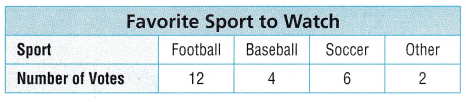A. Start by labeling the bar graph with important information. What will the title of your bar graph be?

Where will you write the label Sport?
Where will you write the scale label Number of Votes?

What labels will the bars of the bar graph have?

The scale for all graphs begins with ___. Since this data set is all even numbers, a scale of ___ is appropriate for this graph.

B. Graph the number of students who voted for each sport by drawing and labeling a bar for each survey answer choice.
The bar labeled Football will end at ____.

The bar labeled Baseball will end at ____.

The bar labeled Soccer will end at ____

The bar labeled Other will end at ___

Draw Conclusions

Use the bar graph you made on the previous page for 1-2.

Question 1.
Why might you use a scale of 2 instead of a scale of 1 for this bar graph?

Question 2.
Explain how using a scale of 4 would change the bar graph.

Share and Show

Use the tables for 1-3.Mathematical Processes
Explain how knowing multiples of numbers helps you choose an appropriate scale for a graph.

Question 1.
A scale of 5 would be more appropriate for a bar graph of the data in the table titled ____.

Question 2.
A scale of 4 would be more appropriate for a bar graph of the data in the table titled ____.

Question 3.
Make bar graphs to display the data in each table.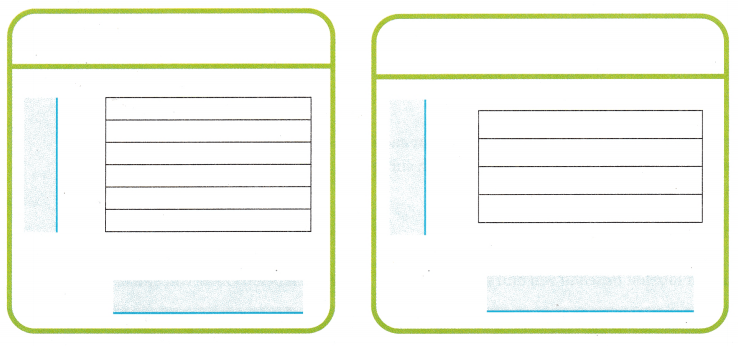Use the bar graph you made on the previous page for 1-2.Question 4.
What’s the Error? Students in Mr. Tran’s class were surveyed about which of four field trip locations they would prefer to visit: an apple orchard, a museum, a planetarium, or a zoo. The results of the survey are shown in the table.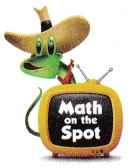Tori made a graph of the data. What error did she make? How can she correct the error?

Question 5.
What scale could Tori have used to make reading the values of the bars easier? Explain.

Question 6.
Multi-Step Exactly how many more students voted for the zoo than the museum? Which data display is easier to use to find the answer? Explain.

Question 7.
Trevor recorded the skateboards that were sold last month in a frequency table. He will display the data in a bar graph. How many bars will the graph have?(A) 3 bars
(B) 4 bars
(C) 10 bars
(D) 8 bars

Use the table and Incomplete graph for 8 and 9.Question 8.
Analyze Suppose you are completing the bar graph. Which scale is best?(A) 1
(B) 2
(C) 12
(D) 10

Question 9.
Multi-Step Brandy graphed the data correctly. Which statement about the graph is true?
(A) The bar for black is twice as tall as the bar for blue.
(B) The bar for black is twice as tall as the bar for red.
(C) The bar for blue is the tallest bar.
(D) The bar for blue is the shortest bar.

Texas Test Prep

Question 10.
Marcy is making a bar graph of the data in the frequency table. What scale should she use?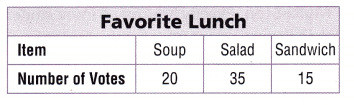(A) 2
(B) 25
(C) 5
(D) 1

### Texas Go Math Grade 5 Lesson 15.3 Homework and Practice Answer Key

Use the tables for 1-3.

Question 1.
A scale of 10 would be more appropriate for a bar graph of the data in the table titled ____.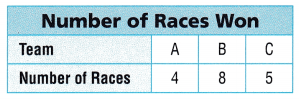Question 2.
A scale of 2 would be more appropriate for a bar graph of the data in the table titled ___.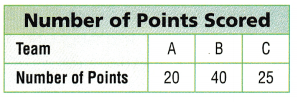Question 3.
Make bar graphs below to display the data in each table.Problem Solving
A pastry chef surveyed her customers to find out which type of berry Is their favorite. The results of the survey are shown in the table and bar graph. Use the table and bar graph for 4-5.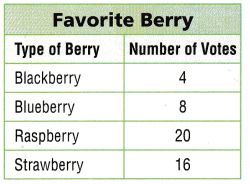Question 4.
What scale was used to make the bar graph? Explain.

Question 5.
Which berry got the most votes? Which data display did you use to find the answer? Explain.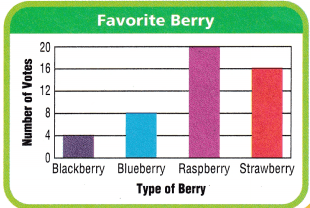Lesson Check

Question 6.
Leon makes a bar graph of the favorite cheese data in the frequency table. How many bars will the graph have?
(A) 12bars
(B) 4 bars
(C) 3 bars
(D) 2 bars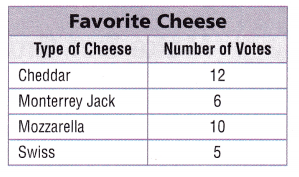Question 7.
Felicia makes a bar graph of the favorite citrus fruit data in the frequency table. Where will the bar for tangerine end?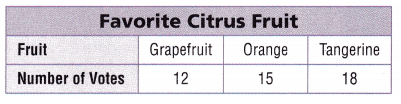(A) 12
(B) 8
(C) 20
(D) 3

Use the table and incomplete graph for 8-10.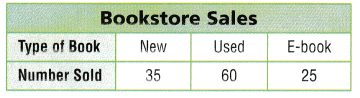Question 8.
Which scale is the best choice for completing the graph?
(A) 5
(B) 2
(C) 4
(D) 1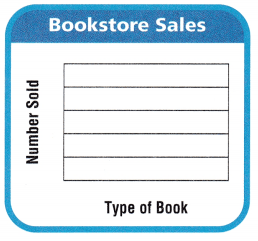Question 9.
Multi-Step The number of hooks the bookstore donates to a library is one fourth the total number of books sold. How many books are donated to a library?
(A) 95
(B) 120
(C) 30
(D) 40